# Python自动化办公之Word批量转成自定义格式的Excel

https://blog.csdn.net/Jacky_kplin/article/details/104367844

python实现word转成自定义格式的excel文档（解决思路和代码）支持按照文件夹去批量处理，也可以单独一个文件进行处理，并且可以自定义标识符。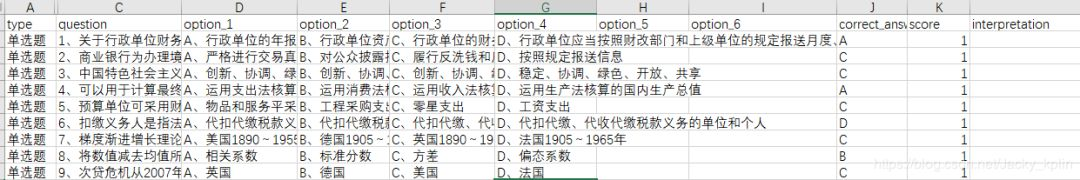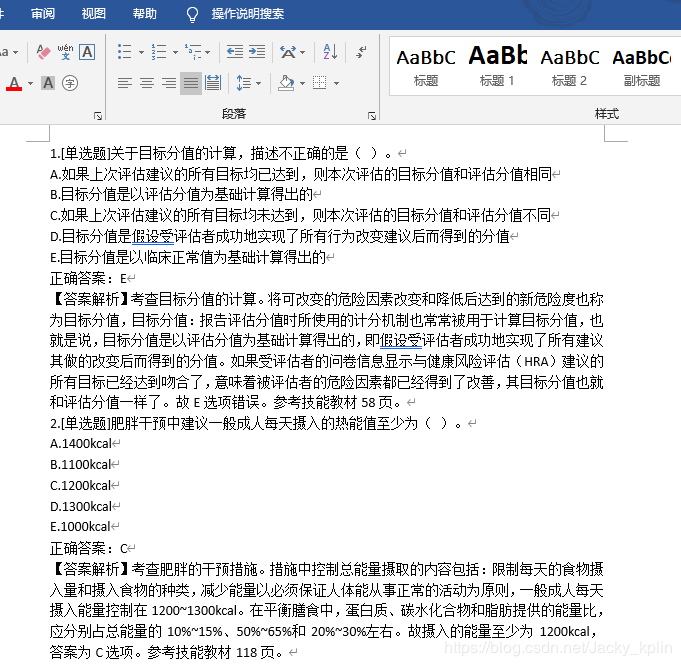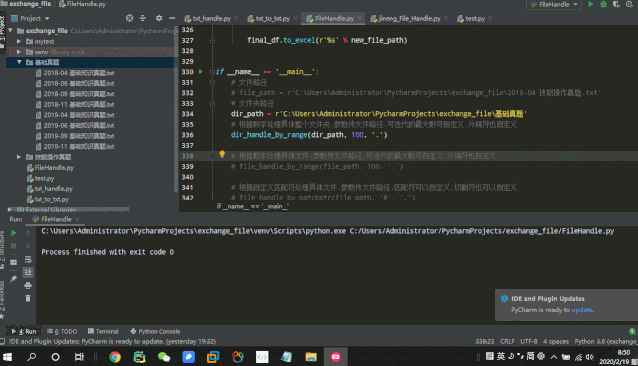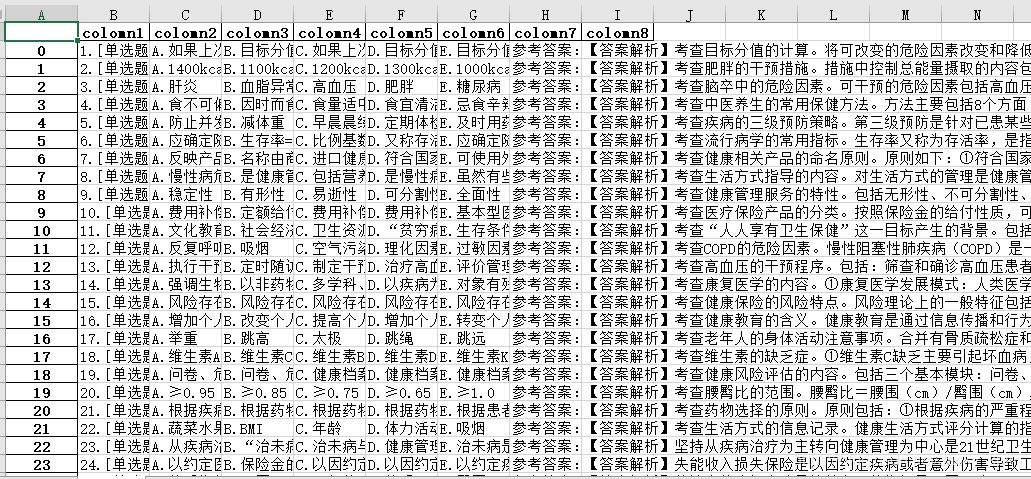import pandas as pd
import os

# 初始处理函数1,先对初始处理结果进行判断
def initail_handle_by_range(file_path, max_page_num, split_str):
# 2、先转成Series
S = pd.Series(df['column1'].values)
# 3、转成列表，列表的每个元素就是每个段落
list = S.tolist()
# 传入一个max_page_num
# 4、遍历列表，取出每个段落，按“.”切割，取出第一个元素进行判断，如果它是题号，就应该得到"1"或者"10"
index_list = []
for content in list:
try:
# 不是每个段落都有“.”可以切割的，会报错，报错就跳过
first_str = content.split('%s'%split_str)
# 5、根据最大的题号，自动生成匹配的字符串，用来匹配题号（每个匹配字符串都放在patch_list中）
patch_list = ['%d' % i for i in range(1, max_page_num + 1)]
# 6、比对切割得到的第一个元素，如果它在匹配的字符串中，就获取它在列表中的索引，并把获取到的结果添加到列表index_list中，这就知道了每道题的开头在l中的哪个位置了
if first_str in patch_list:
index = list.index(content)
index_list.append(index)
except:
pass
# 7、根据索引列表，我们可以知道每道题的第一段和最后一段在l中的哪个位置
# index_list = [0, 8, 16]
# print(index_list)
# 先计算每道题长度是否一致,不一致的,设置is_same_length = False
is_same_length = True
length = index_list - index_list
max_index = len(list)
for i in index_list:
# 如果i不是最后一个，那么start就是i，end就是i的下一个
if i < index_list[-1]:
start = i
end = index_list[index_list.index(i) + 1]
else:
start = i
end = max_index
# 判断长度是否一致,不一致就对长度进行比较,把大的赋值给长度
if (end - start) != length:
is_same_length = False
if (end - start) > length:
length = (end - start)

result = [is_same_length, index_list, list, length]

return result

# 初始处理函数1,先对初始处理结果进行判断
def initail_handle_by_patchstr(file_path, patch_str, split_str):
# 2、先转成Series
S = pd.Series(df['column1'].values)
# 3、转成列表，列表的每个元素就是每个段落
list = S.tolist()
# 传入一个max_page_num
# 4、遍历列表，取出每个段落，按“.”切割，取出第一个元素进行判断，如果它是题号，就应该得到"1"或者"10"
index_list = []
for content in list:
try:
# 不是每个段落都有“.”可以切割的，会报错，报错就跳过
first_str = content.split('%s'%split_str)
# 6、比对切割得到的第一个元素，如果它在匹配的字符串中，就获取它在列表中的索引，并把获取到的结果添加到列表index_list中，这就知道了每道题的开头在l中的哪个位置了
if first_str == patch_str:
index = list.index(content)
index_list.append(index)
except:
pass
# 7、根据索引列表，我们可以知道每道题的第一段和最后一段在l中的哪个位置
# index_list = [0, 8, 16]
# print(index_list)
# 先计算每道题长度是否一致,不一致的,设置is_same_length = False
is_same_length = True
length = index_list - index_list
max_index = len(list)
for i in index_list:
# 如果i不是最后一个，那么start就是i，end就是i的下一个
if i < index_list[-1]:
start = i
end = index_list[index_list.index(i) + 1]
else:
start = i
end = max_index
# 判断长度是否一致,不一致就对长度进行比较,把大的赋值给长度
if (end - start) != length:
is_same_length = False
if (end - start) > length:
length = (end - start)

result = [is_same_length, index_list, list, length]

return result

# 传入一个文件路径和一个匹配的字符串,至少2个符号,例如"#."
def file_handle_by_patchstr(file_path, patch_str, split_str):
result = initail_handle_by_patchstr(file_path, patch_str, split_str)
# 接收初始处理函数的结果
is_same_length = result
index_list = result
list = result
length = result
# 先根据每道题的长度构造一个空的dict，最后用来生成dataFrame
dict = {}
for i in range(1, (length + 1)):
dict['colomn%d' % i] = []
# print(dict)
max_index = len(list)
for i in index_list:
# 如果i不是最后一个，那么start就是i，end就是i的下一个
if i < index_list[-1]:
start = i
end = index_list[index_list.index(i) + 1]
else:
start = i
end = max_index
# 遍历一轮获得的结果就是我们要写入excel的一行
colomn = 1
for index in range(start, end):
# 遍历一次获得的结果就是我们要写入excel的一格
content = list[index]
# 每遍历一次就在一个dict中取出某一列，给它加上这个数据
dict['colomn%d' % colomn].append(content)
colomn += 1
# 在遍历到最后一轮的时候
if index == (end - 1):
# 判断是否需要插入空值
if not is_same_length and ((end - start) < length):
# 可能缺了2列,也可能缺了1列
content = ''
# 如果只是缺了一列,在现有的index上,加1,就是下一列,补上空值
if (length - (end - start)) == 1:
colomn = (end - start) + 1
dict['colomn%d' % (colomn)].append(content)
else:
# 如果缺失了2列以上,就遍历,给之后的每一列都补上空值
for i in range(length - (end - start)):
colomn = length - i
dict['colomn%d' % (colomn)].append(content)

final_df = pd.DataFrame(dict)

new_file_path = file_path.split('.') + '.xlsx'

final_df.to_excel(r'%s' % new_file_path)

def file_handle_by_range(file_path, max_page_num, split_str):
result = initail_handle_by_range(file_path, max_page_num, split_str)
# 接收初始处理函数的结果
is_same_length = result
index_list = result
list = result
length = result

# 先根据每道题的长度构造一个空的dict，最后用来生成dataFrame
dict = {}
for i in range(1,(length+1)):
dict['colomn%d'%i] = []
max_index = len(list)
for i in index_list:
# 如果i不是最后一个，那么start就是i，end就是i的下一个
if i < index_list[-1]:
start = i
end = index_list[index_list.index(i) + 1]
else:
start = i
end = max_index
# 遍历一轮获得的结果就是我们要写入excel的一行
colomn = 1
for index in range(start, end):
# 遍历一次获得的结果就是我们要写入excel的一格
content = list[index]
# 每遍历一次就在一个dict中取出某一列，给它加上这个数据
dict['colomn%d' % colomn].append(content)
colomn += 1
# 在遍历到最后一轮的时候
if index == (end - 1):
# 判断是否需要插入空值
if not is_same_length and ((end-start)<length):
# 可能缺了2列,也可能缺了1列
content = ''
# 如果只是缺了一列,在现有的index上,加1,就是下一列,补上空值
if (length-(end-start)) == 1:
colomn = (end-start) +1
dict['colomn%d'% (colomn)].append(content)
else:
# 如果缺失了2列以上,就遍历,给之后的每一列都补上空值
# 8-6 = 2, i = 0, 1
for i in range(length-(end-start)):
colomn = length - i
dict['colomn%d' % (colomn)].append(content)
final_df = pd.DataFrame(dict)

new_file_path = file_path.split('.') + '.xlsx'

final_df.to_excel(r'%s'%new_file_path)

# 传入一个文件夹路径，最大的迭代数字，切割字符串
def dir_handle_by_range(dir_path, max_page_num, split_str):
files = os.listdir(dir_path)  # 获取当前目录的所有文件及文件夹
for file in files:
file_path = os.path.join(dir_path, file)  # 遍历获取每个文件的绝对路径
result = initail_handle_by_range(file_path, max_page_num, split_str)
# 接收初始处理函数的结果
is_same_length = result
index_list = result
list = result
length = result

# 先根据每道题的长度构造一个空的dict，最后用来生成dataFrame
dict = {}
for i in range(1, (length + 1)):
dict['colomn%d' % i] = []
max_index = len(list)
for i in index_list:
# 如果i不是最后一个，那么start就是i，end就是i的下一个
if i < index_list[-1]:
start = i
end = index_list[index_list.index(i) + 1]
else:
start = i
end = max_index
# 遍历一轮获得的结果就是我们要写入excel的一行
colomn = 1
for index in range(start, end):
# 遍历一次获得的结果就是我们要写入excel的一格
content = list[index]
# 每遍历一次就在一个dict中取出某一列，给它加上这个数据
dict['colomn%d' % colomn].append(content)
colomn += 1
# 在遍历到最后一轮的时候
if index == (end - 1):
# 判断是否需要插入空值
if not is_same_length and ((end - start) < length):
# 可能缺了2列,也可能缺了1列
content = ''
# 如果只是缺了一列,在现有的index上,加1,就是下一列,补上空值
if (length - (end - start)) == 1:
colomn = (end - start) + 1
dict['colomn%d' % (colomn)].append(content)
else:
# 如果缺失了2列以上,就遍历,给之后的每一列都补上空值
for i in range(length - (end - start)):
colomn = length - i
dict['colomn%d' % (colomn)].append(content)

final_df = pd.DataFrame(dict)

new_file_path = file_path.split('.') + '.xlsx'

final_df.to_excel(r'%s' % new_file_path)

# 传入一个文件夹路径和一个匹配的字符串,至少2个符号,例如"#."
def dir_handle_by_patchstr(dir_path, patch_str, split_str):
files = os.listdir(dir_path)  # 获取目录的所有文件及文件夹
for file in files:
file_path = os.path.join(dir_path, file)  # 遍历获取每个文件的绝对路径
print(file_path)
result = initail_handle_by_patchstr(file_path, patch_str, split_str)
# 接收初始处理函数的结果
is_same_length = result
index_list = result
list = result
length = result
# 先根据每道题的长度构造一个空的dict，最后用来生成dataFrame
dict = {}
for i in range(1, (length + 1)):
dict['colomn%d' % i] = []
# print(dict)
max_index = len(list)
for i in index_list:
# 如果i不是最后一个，那么start就是i，end就是i的下一个
if i < index_list[-1]:
start = i
end = index_list[index_list.index(i) + 1]
else:
start = i
end = max_index
# 遍历一轮获得的结果就是我们要写入excel的一行
colomn = 1
for index in range(start, end):
# 遍历一次获得的结果就是我们要写入excel的一格
content = list[index]
# 每遍历一次就在一个dict中取出某一列，给它加上这个数据
dict['colomn%d' % colomn].append(content)
colomn += 1
# 在遍历到最后一轮的时候
if index == (end - 1):
# 判断是否需要插入空值
if not is_same_length and ((end - start) < length):
# 可能缺了2列,也可能缺了1列
content = ''
# 如果只是缺了一列,在现有的index上,加1,就是下一列,补上空值
if (length - (end - start)) == 1:
colomn = (end - start) + 1
dict['colomn%d' % (colomn)].append(content)
else:
# 如果缺失了2列以上,就遍历,给之后的每一列都补上空值
for i in range(length - (end - start)):
colomn = length - i
dict['colomn%d' % (colomn)].append(content)
final_df = pd.DataFrame(dict)
print(final_df)
print(file_path)

new_file_path = file_path.split('.') + '.xlsx'
print(new_file_path)

final_df.to_excel(r'%s' % new_file_path)

if __name__ == '__main__':
# 文件路径
# 文件夹路径

# 根据数字处理具体文件,参数传文件路径,可迭代的最大数可自定义,分隔符也自定义
# file_handle_by_range(file_path, 100, '.')

# 根据自定义匹配符处理具体文件,参数传文件路径,匹配符可以自定义,切割符也可以自定义
# file_handle_by_patchstr(file_path, '#', '.')

# 根据数字处理具体整个文件夹,参数传文件路径,可迭代的最大数可自定义,分隔符也自定义
# dir_handle_by_range(dir_path, 100, '.')

# 根据自定义匹配符处理具体文件夹,参数传文件路径,匹配符可以自定义,切割符也可以自定义
# dir_handle_by_patchstr(dir_path, '#', '.')


1、python怎么读取这个文件的内容？

python有相关的word操作库可以读取，但是读取到的结果不方便操作。我们最终是要让它生成excel文件的，所以可以用python非常强大的科学计算包pandas来读取操作数据更好。2、读取到的数据如何处理？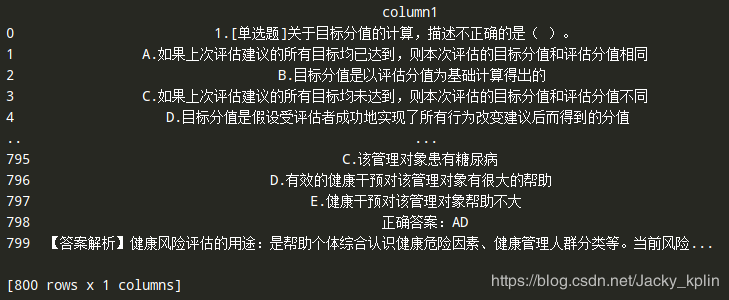3、接下来怎么处理呢？

pandas强大的地方就在这里了，它可以直接把这1列的内容全部转成Series，Series是什么你可以不用管，你只需要知道Series可以直接转成列表list就行了。有了list我们就方便操作了。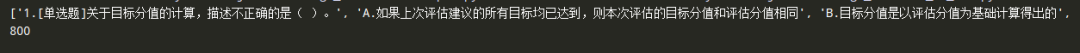1、你的word文档结构相对合理些，每道题都固定是X个段落（例如每道题都是8个段落），这个解决就很简单了。因为这种情况对应就是在list中，每8个元素构成了1道题，你只要按照规律，8个8个取出，最后批量写入excel就行了。（这种解决起来比较简单，就不详细说了）

2、另一种情况比较麻烦，就是word文档的结构不规范，有的题目可能是8个段落，有的可能是7段，有的是6段。那么要怎么解决呢？

1、excel表格是行列结构的，它每一行的数据来源于这个txt文档的第几行？（也就是list里面的第几个元素）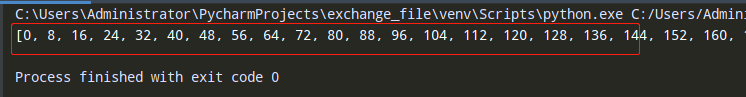0代表了第一道题是在列表的第1个元素开始的，8代表第二道题在列表的第9个元素开始。

2、拿到了每道题的索引，然后怎么做呢？

3、问题的关键就变成了，有了每道题的索引，怎么把它转成dataFrame结构。

dict = {'colomn1': ['问题1', '问题2', '问题3'],
'colomn2': ["A：问题1的A选项", "A：问题2的A选项", "A：问题3的A选项"],
'colomn3': ['B：问题1的B选项', 'B：问题2的B选项', 'B：问题3的B选项']}

df = pd.DataFrame(dict)

print(df)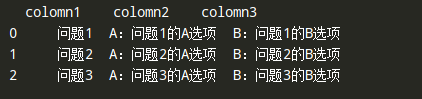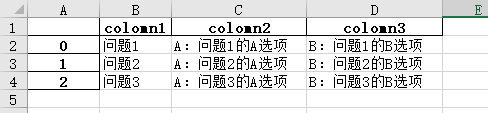dict = {'colomn1': ['问题1', '问题2', '问题3'],
'colomn2': ["A：问题1的A选项", "A：问题2的A选项", "A：问题3的A选项"],
'colomn3': ['B：问题1的B选项', 'B：问题2的B选项', 'B：问题3的B选项']}



list = [‘问题1’,“A：问题1的A选项”, ‘B：问题1的B选项’…‘问题2’,“A：问题2的A选项”, ‘B：问题2的B选项’]

for i in range(start, end):
content = list[i]


1、python解释器（pycharm或其他）；

2、python自带模块：os；

3、自行安装模块：pandas，openpyxl；

1、先要做数据预处理：先要把word文档的内容复制到txt文档中，并且在第一行加上"column1"，处理后像下面这样：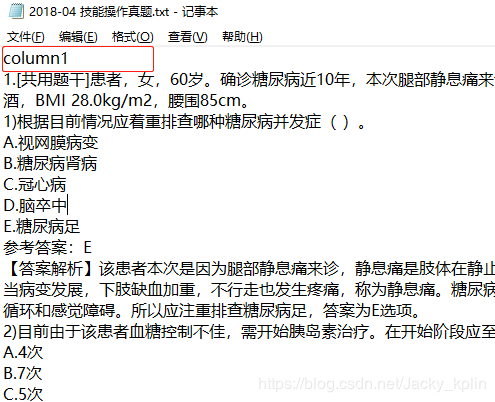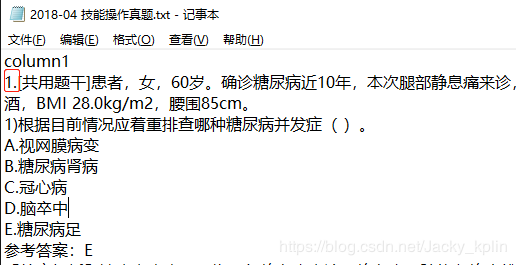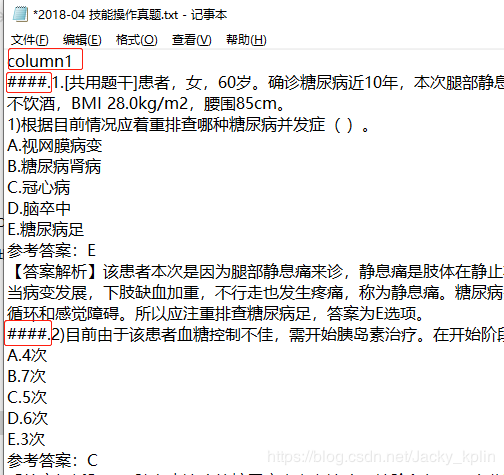file_handle_by_patchstr(file_path, '####', '.')


2、可调用的有4个函数：

2.1、假如你只想处理一个具体的文档，并且它有具体的题号，最大题号是100，并且它后面跟的是一个“.”，那么就按照下面这个调用

file_path = r'C:\Users\Administrator\PycharmProjects\exchange_file\2018-04 技能操作真题.txt'
file_handle_by_range(file_path, 100, '.')


2.2、根据自定义匹配符处理具体文件,参数传文件路径,匹配符可以自定义,切割符也可以自定义

file_path = r'C:\Users\Administrator\PycharmProjects\exchange_file\2018-04 技能操作真题.txt'
file_handle_by_patchstr(file_path, '#', '.')


2.3、根据数字处理具体整个文件夹,参数传文件路径,可迭代的最大数可自定义,分隔符也自定义。（注意文件夹路径，最后是不跟文件名的，它是个文件夹，不是具体文件路径）

# 文件夹路径
dir_handle_by_range(dir_path, 100, '.')


2.4、根据自定义匹配符处理具体文件夹,参数传文件路径,匹配符可以自定义,切割符也可以自定义

# 文件夹路径
dir_handle_by_patchstr(dir_path, '#', '.')


< END >07-22449804-21951
02-183698
07-04146
09-301829
05-091万+
04-21241
04-115301
08-277758